# How to create a mosaic plot in Excel

Excel
Pie and bar charts greatly simplify the understanding of percentages distribution for one categorical variable but fail to build a meaningful representation of two and more variables. A quite recent innovation in data visualization real is the Mosaic plot, which helps to grasp the correlations within marketing, sales, and other financial data. You will not find this chart among Excel standard charts, but you can build one.

The Mosaic plot, also referenced as Marimekko or Mekko chart, and the market map used to visualize, for example:

• The percentage of the data for the primary and secondary categories. Such as the ratio of men and women for the crew and each class of passengers:The Mosaic chart is a two-dimensional mixture of both a 100% stacked column and a 100% stacked bar chart in Excel. The crucial feature is the variable column width and height that corresponds to the fraction along vertical and horizontal dimensions.

To create a mosaic plot in Excel, you need to follow the below steps (not so easy to guess though):

1.   Re-shape the data into simple data series.

2.   Create a step area chart (see How to create a step Area chart for the Mosaic plot in Excel).

3.   Add labels to the chart (see How to add labels to the mosaic plot).

For example, for the Casualties and survivors of the Titanic tragedy: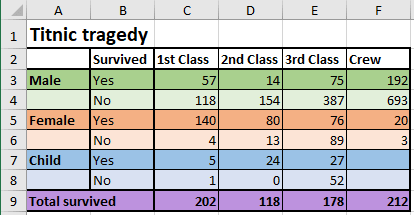To build a mosaic plot in Excel, you need to re-shape the data into simple data series.

The Area chart creates a mosaic effect by varying sub-areas width and heights.

To create an area chart, first, create a table containing widths in the first column of each row and the heights in the following item columns: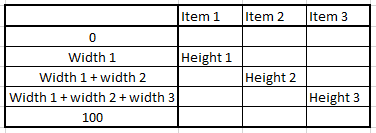1.   Calculate the height for each row of the chart:

The height for the first row of items is:

= C9 / SUM (C9:F9), = 202 / (202 + 118 + 178 + 212) = 28.45%,

The height of all the following rows are:

= D9 / SUM (C9:F9), = 118 / (202 + 118 + 178 + 212) = 16.62%,

= E9 / SUM (C9:F9), = 178 / (202 + 118 + 178 + 212) = 25.07%,

= F9 / SUM (C9:F9), = 212 / (202 + 118 + 178 + 212) = 29.86%.

2.   Calculate the X values for the first row of the chart, starting from 0:

= C7 / C9 * 100 = 2 – the percent of survived children of all survived in the 1st class,

= (C7 + C5) / C9 * 100 = 72 – the percent of survived children and women of all survived in the 1st class,

= 100 as 100% of survived in the 1st class: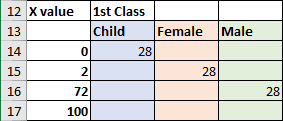3.   Calculate the X values for the second row in the chart:

= D7 / D9 * 100 = 20 – percent of survived children of all survived in the 2nd class,

= (D7 + D5) / D9 * 100 = 88 – percent of survived children and women of all survived in the 2nd class.

4.   Calculate the X values for the third row in the chart:

= E7 / E9 * 100 = 15 – percent of survived children of all survived in the 3rd class,

= (E7 + E5) / E9 * 100 = 58 – percent of survived children and women of all survived in the 3rd class.

5.   Calculate the X values for the last row in the chart:

= F5 / F9 * 100 = 9 – percent of survived women of survived crew members.

So, for this example, the X values are:

0, 2, 72, 100 and 20, 88 and 15, 88 and 9.

6.   Insert the X values in sorted order into the table and add up the appropriate heights: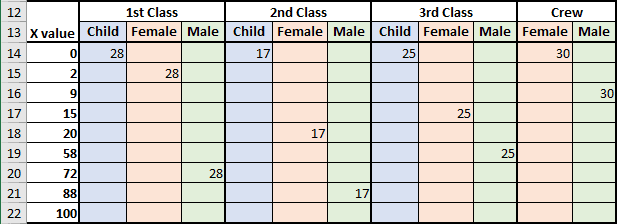7.   To create a step chart, you will need two copies of the same values:

• the start value (previous value)
• the end value (current value).

For this example: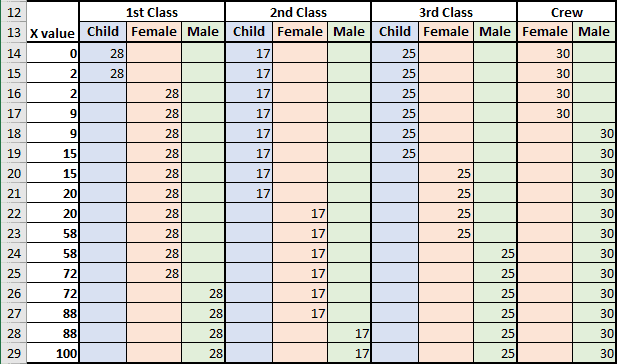The data for the step Area chart is prepared. For the following step, see How to create a step Area chart for the Mosaic plot in Excel.

If you have any questions or suggestions, please feel free to ask OfficeToolTips team.

We use cookies to personalise content and ads, to provide social media features and to analyse our traffic. We also share information about your use of our site with our social media, advertising and analytics partners who may combine it with other information you’ve provided to them or they’ve collected from your use of their services.